# Higher-Order Functions

Episode #5 • Feb 26, 2018 • Subscriber-Only

Most of the time we interact with code we did not write, and it doesn’t always play nicely with the types of compositions we have developed in previous episodes. We explore how higher-order functions can help unlock even more composability in our everyday code.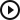Higher-Order Functions
Introduction
00:11
Curry
00:42
Flip
04:15
Unbound methods
07:18
A problem
10:18
Zurry
12:42
Higher-order
14:32
What’s the point?
20:39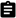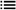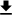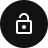### Introduction

Today we’re going to dive a bit deeper into function composition. While function composition can seem simple, it’s not always so easy to take the existing functions we work with every day and fit them into our compositions. Let’s explore some reusable techniques that can aid us in transforming functions that are difficult to compose into pieces that fit snugly.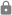This episode is for subscribers only.

### Subscribe to Point-Free

Access this episode, plus all past and future episodes when you become a subscriber.

See plans and pricing

### Exercises

1. Write `curry` for functions that take 3 arguments.

Solution
``````func curry<A, B, C, Z>(
_ f: @escaping (A, B, C) -> Z
) -> (A) -> (B) -> (C) -> Z {

return { a in { b in { c in f(a, b, c) } } }
}
``````
2. Explore functions and methods in the Swift standard library, Foundation, and other third party code, and convert them to free functions that compose using `curry`, `zurry`, `flip`, or by hand.

3. Explore the associativity of function arrow `->`. Is it fully associative, i.e. is `((A) -> B) -> C` equivalent to `(A) -> ((B) -> C)`, or does it associate to only one side? Where does it parenthesize as you build deeper, curried functions?

Solution

If the function `((A) -> B) -> C` were equivalent to `(A) -> ((B) -> C)` then we’d be able to write a function that can transform from one to the other. For example, we would be able to implement this function:

``````func equivalence<A, B, C>(
_ f: @escaping (A) -> ((B) -> C)
) -> ((A) -> B) -> C {
return { f in
// How to return something in C in here???
}
}
``````

However, it is not possible to implement this function.

It turns out, the function arrow `->` only associates to the right. So if we were to write:

``````f: (A) -> (B) -> (C) -> D
``````

what that really means is:

``````f: (A) -> ((B) -> ((C) -> D))
``````
4. Write a function, `uncurry`, that takes a curried function and returns a function that takes two arguments. When might it be useful to un-curry a function?

5. Write `reduce` as a curried, free function. What is the configuration vs. the data?

Solution

The reduce method on collections takes two arguments: the initial value to reduce into, and the accumulation function that takes what has been accumulated so far and a value from the array and must return a new accumulation value. The accumulation function is most like configuration in that it describes how to perform the reduce, and is most likely to be reused across many reduces. Whereas the initial value, and the collection being operated on, are like the data since it’s what you aren’t likely to have access to until the moment you want to reduce. So a possible curried signature of `reduce` might take the accumulation upfront, and delay the initial value and collection:

``````func reduce<A, R>(
_ accumulator: (R, A) -> R
) -> (R) -> ([A]) -> R {

return { initialValue in
return { collection in
return collection.reduce(initialValue, accumulator)
}
}
}
``````
6. In programming languages that lack sum/enum types one is tempted to approximate them with pairs of optionals. Do this by defining a type `struct PseudoEither<A, B>` of a pair of optionals, and prevent the creation of invalid values by providing initializers.

This is “type safe” in the sense that you are not allowed to construct invalid values, but not “type safe” in the sense that the compiler is proving it to you. You must prove it to yourself.

Solution

The `PseudoEither` type could be defined as:

``````struct PseudoEither<A, B> {
let left: A?
let right: B?

init(left: A) {
self.left = left
self.right = nil
}

init(right: B) {
self.left = nil
self.right = right
}
}
``````

It is not possible to construct values of `PseudoEither` where both `left` and `right` hold some value, but nevertheless it is not the compiler proving this but rather you having to make sure this remains true. We talk more about this in our episode on algebraic data types.

7. Explore how the free `map` function composes with itself in order to transform a nested array. More specifically, if you have a doubly nested array `[[A]]`, then `map` could mean either the transformation on the inner array or the outer array. Can you make sense of doing `map >>> map`?

#### Everything’s a Function.

Eitan Chatav • Friday Jan 18, 2013

This short article explains how everything can be seen to be a function, even values and function application. Eitan coins the term `zurry` to describe the act of currying a zero-argument function.